• 欢迎您访问河南成考网，本站为民间交流网站！  今天是2020年05月30日星期六
• 河南成考网提供河南各院校成考辅导报名，咨询电话：

# 2016年高起点理工农医类数学考试真题及答案

2018-08-10 19:15:43   来源：河南成考网    点击：

一、选择题：本大题共17小题，每小题5分，共85分。在每小题给出的四个选项中，只有一项是符合题目要求的。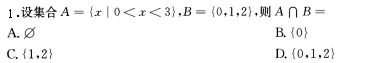答案：C

2.函教y=2sinxcosx的最小正周期是()

A.π/2 B.π C.2π D.4π

答案：B

3.等差数列{an)中，若a1=2，a3=6，则a7=()

A.14 B.12 C.10 D.8

答案：A

4.将一颗骰子抛掷1次，则得到的点教为偶数的概率为()

A.2/3 B.1/2 C.1/3 D.1/6

答案：B

5.不等式|2x-3|<1的解集为()

A.{x|1

B.{x|x<-l 或 x>2}

C.{x|1

D.{x|2

答案：C

6-下列函数中，为偶函教的是()

A.y=log2x B.y=X2+X C.y=4/x D.y=x2

答案：D

7.若tanα=3,则tan(α+π/4)=

A.2 B.1/2 C.-2 D.-4

答案：C

8.642/3+log1/981=

A.8 B.10 C.12 D.14

答案：D

9.曲线y=x2+l与直线y=2x的交点坐标为( )

A.(1.2) B.(-1，2) C.(2，4)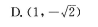答案：A

10.已知正六棱锥的底面边长为3，侧棱长为5，则该六棱锥的体积为()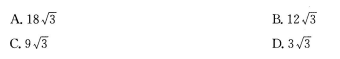答案：A

11.过点(0，1)且与直线x+y+1=0垂直的直线方程为()

A.y=x B.y=2x+l C.y=x+1 D.y=x-l

答案：C

12.设双曲线x2/16-y2/9=1的渐近线的斜率为k，则|k|=

答案:B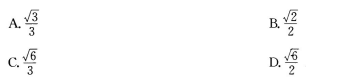答案：A

14.若函数y=(αx+1)/(2x-3)的图像与其反函数的图像重合，则α=

A.-3 B.1 C.2 D.3

答案：D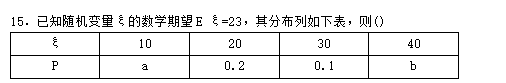A.a=0.4，b=0.3

B.a=0.3，b=0.4

C.a=0.2，b=0.5

D.a=0.5，b=0.2

答案：A

16.在(l+2x)8的展开式中，22的系数为()

A.16

B.28

C.56

D.112

答案：D

17.曲线y=x3-4x+2在点(1，-1)处的切线方程为()

A.x-y-2=0

B.x-y=0

C.x+y=0

D.27+y-2=0

答案：C

二、填空题：本大题共4小题，每小题4分，共16分，把答案填在题中横线上。

18.若平面向量a=(x,1),b=(1,-2),且a//b，则x=

答案：-1/2

19.若二次函数f(x)=ax2+2x的最小值为-1/3，则a=

答案：3

20.复数(2-2i)/(1+i)2的模为

答案：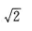21.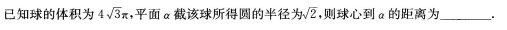答案：1

三、解答题：本大题共有4小题，共49分。解答应写出推理、演算步骤。

22.(本小题满分12分)

在△ABC中，AB=2，BC=3，B=60°。求AB及△ABC的面积。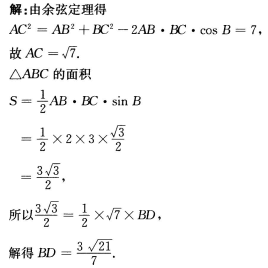23.(本小题满分12分)

已知等比数列{an}的各项都是正数，且a1+a3=10,a2+a3=6。

(1)求{an}的通项公式;

(2)求{an}的前5项和。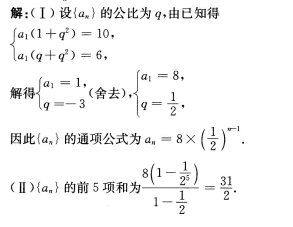24.(本小题满分12分)25.(本小题满分13分)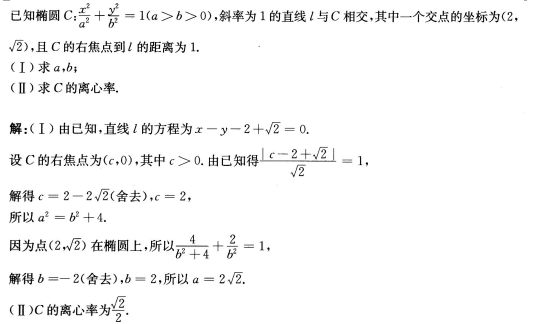#### 【考试时间：10月24、25日】

【请广大考生提前备考】
##### 加入河南成考公众号河南成考网

(扫一扫加入)
##### 加入河南成考交流群